# Continued Fractions

A neat way to represent a number is as a continued fraction. A simple continued fraction is a fraction that looks like this:

a1 + 1/(a2 + 1/(a3 + 1/(a4 + 1/(...))))

This gives a better view of it, but it’s harder to type: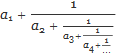They’re called “simple” continued fractions because all of the intermediate numerators are 1.

A more compact notation for a simple continued fraction is [a1; a2, a3, a4, ...]. The semicolon after a1 is intentional; separating the integer part from the rest of the terms. (Donald Knuth uses an attractive notation that looks kind of like //a1, a2, a3, ...//, but no one else seems to use it.) Continued fractions have many interesting properties that we won’t get to today.

For a simple example, take 7/2. Expressed as a continued fraction, it’s 3 + 1/2. All of the numerators are 1, so we’re done. In compact notation, it’s [3; 2].

Slightly trickier, take 2/7. When a numerator isn’t 1, a general procedure is to take the reciprocal of that part: 2/7 is the same as 1/(7/2). Now the numerator is how we want it, but the denominator isn’t. If we split the denominator into integer and fractional parts, though, we get 1/(3 + 1/2), which is the shape we want. The compact form is [0; 3, 2]. (In this compact notation, the only difference between reciprocals is that one of them has that zero in front.)

There’s a nice, sort-of-graphical way to compute the continued fraction for a rational number. Take a fraction, say, 22/11. A way to visualize this is to make a 22×11 rectangle, and then fill it with as many of the largest square you can fit. In this case, the largest square that will fit is 11×11. The denominator 11 exactly divides 22, so you can completely fill the rectangle with 2 of those squares. That gives the final result of 2, or  if you use the compact continued fraction notation.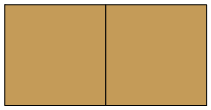So what about 23/11? Given a 23×11 rectangle, you can fit two 11×11 squares, but there’s that piece left over. So we know it’s 2 plus something.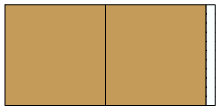The leftover strip is 1 unit wide by 11 units tall, and you can fill it with eleven 1×1 squares, so the fraction 23/11 is equal to 2 + 1/11. The rectangle is completely filled, and the continued fraction notation is [2; 11].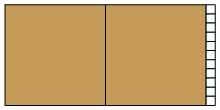Okay, how about 24/11? You can still fit the two 11×11 squares, leaving a 2×11 strip, so it’s 2 + 2/11.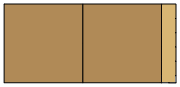If you tilt your head sideways while looking at the leftover strip, an equivalent total is 2 + 1/(11/2), plus we can now break up the strip into smaller squares as before. Specifically, you can fit five 2×2 squares into the strip, leaving a little left over, a 2×1 strip. This means 11/2 is the same as 5 + 1/2, making the original fraction 24/11 equal to 2 + 1/(5 + 1/2).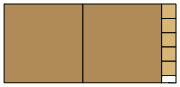Finally, that last 2×1 strip can be divided into 1×1 squares. Counting up the rectangles, we get [2; 5, 2].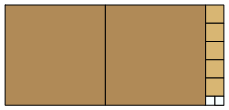Continuing, 25×11 takes two 11×11 squares plus a 3×11 strip, so 25/11 = 2 + 3/11 = 2 + 1/(11/3).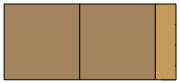Filling the 3×11 strip with three 3×3 squares plus leftovers gives 3 + 2/3, making the original 25/11 equal to 2 + 1/(3 + 2/3). Anticipating the next step, that’s the same as 2 + 1/(3 + 1/(3/2)).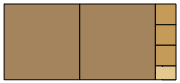That remaining 3×2 strip can be filled by one 2×2 square, leaving a 2×1 strip, giving us 2 + 1/(3 + 1/(1 + 1/2)).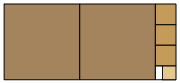The final 2×1 strip takes two 1×1 squares, finishing the process.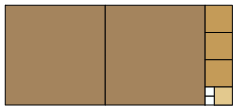After all that, 25/11 = 2 + 1/(3+ 1/(1 + 1/2)), or [2; 3, 1, 2].

Continuing the pattern, 26/11 through to 33/11 look like this.

26/11 = [2; 2, 1, 3]: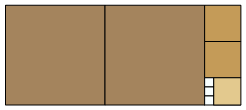27/11 = [2; 2, 5]: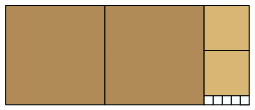28/11 = [2; 1, 1, 5]: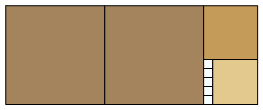29/11 = [2; 1, 1, 1, 3]: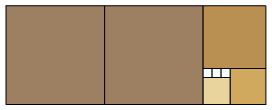30/11 = [2; 1, 2, 1, 2]: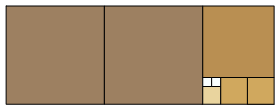31/11 = [2; 1, 4, 2]: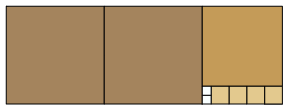32/11 = [2; 1, 10]: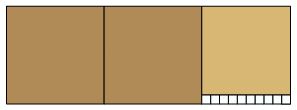33/11 = :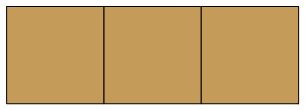This graphical procedure is essentially Euclid’s algorithm for computing greatest common divisors: the side of the final square used to fill the rectangle is the GCD of the numerator and denominator. For 22/11, the final square’s side was 11, and GCD(22, 11) = 11. For 23/11, the final square had side 1, so GCD(23, 11) = 1. And so on. From this procedure we can tell that rational numbers have finite continued fraction representations.

Ratios of successive Fibonacci numbers have the longest continued fraction representations, and look the closest to a spiral using the graphical procedure. Here are 3/2 = [1; 2], 5/3 = [1; 1, 2], 8/5 = [1; 1, 1, 2], 13/8 = [1; 1, 1, 1, 2], 21/13 = [1; 1, 1, 1, 1, 2], 34/21 = [1; 1, 1, 1, 1, 1, 2], 55/34 = [1; 1, 1, 1, 1, 1, 1, 2].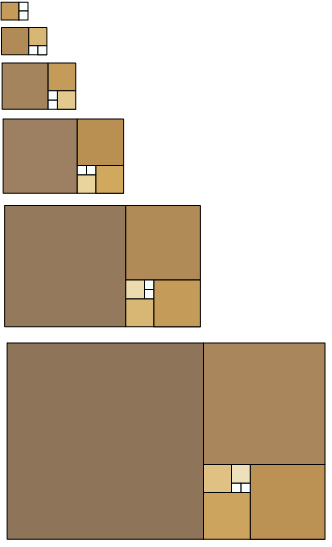It turns out that irrational numbers have never-ending continued fraction representations. The golden ratio, which is the limit of the ratio of consecutive Fibonacci numbers, is [1; 1, 1, 1, 1, 1, ...]. Quadratic irrationals have repeating representations: The square root of 2 is [1;2,2,2,2,2,...] and the square root of 3 is [1;1,2,1,2,1,2,...]. Some of our favorite numbers have interesting patterns: e is [2;1,2,1,1,4,1,1,6,1,1,8,1,1,10,1,...].

Designed and rendered using Mathematica 9.